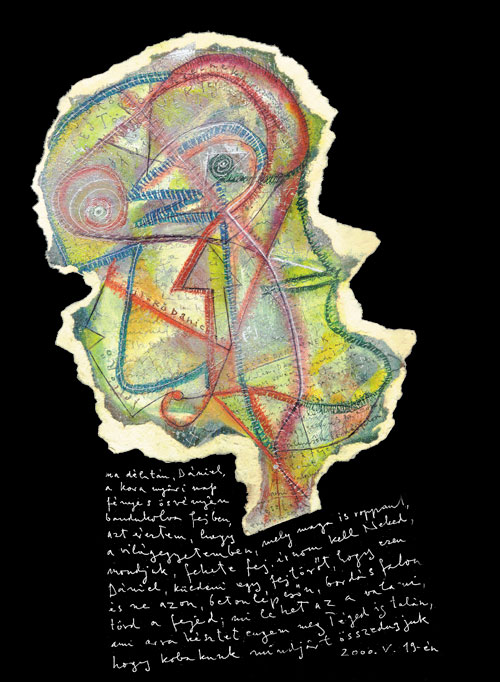FejtÃ¶rÅ‘, DÃ¡nielnek

M A Â D Ã‰ L U T Ã N, D Ã N I E L, A Â K O R A Â N Y Ã R I

N A P Â F Ã‰ N Y E S Â Ã– S V Ã‰ N Y Ã‰ N Â B A N D U K O L V A

F E J B E N, Â A Z T Â Ã‰ R E Z T E M, Â H O G Y Â A Â V I L Ã G-

E G Y E T E M B E N, Â M E L Y Â M A G A Â I S Â R O P P A N T,

M O N D J U K, Â F E K E T E Â F E J, Â Ã R N O M Â K E L L

N E K E D, Â D Ã N I E L, K Ãœ L D E N I Â E G Y Â F E J-

T Ã– R Å T, Â H O G Y Â E Z E N Â Ã‰S Â N E Â A Z O N,

B E T O N L Ã‰ P C S Å N, Â B O R D Ã S F A L O N

T Ã– R D Â A Â F E J E D; Â M I Â L E H E T

A Z Â A Â V A L A M I, Â A M I Â A R R A Â K Ã‰ S Z T E T,

E N G E M Â M E G Â T Ã‰ G E D Â I S Â T A L Ã N,

H A Â V Ã‰ G Ã‰ H E Z Â Ã‰ R Â E Z Â A Â D Ã‰ L U T Ã N

Ã‰ S Â T A L Ã L K O Z U N K, Â H O G Y Â K O B A K U N K

M I N D J Ã R T Â Ã– S S Z E D U G J U K Â S Â N E Â C S A K

F E J B E N, Â D E Â V A L Ã“ BA N, Ã‰ S Â Ã‰ R E Z Z Ãœ K,

H O G Y Â A Â H I B B A N T Â V I L Ã G B AN

E Z Â J Ã“ L Â V A N; Â N O S, M I Â E Z Â A Â M I ?

M O N D D Â M E G, Â H A Â K I T A L Ã L T A D, D A N I.

Â